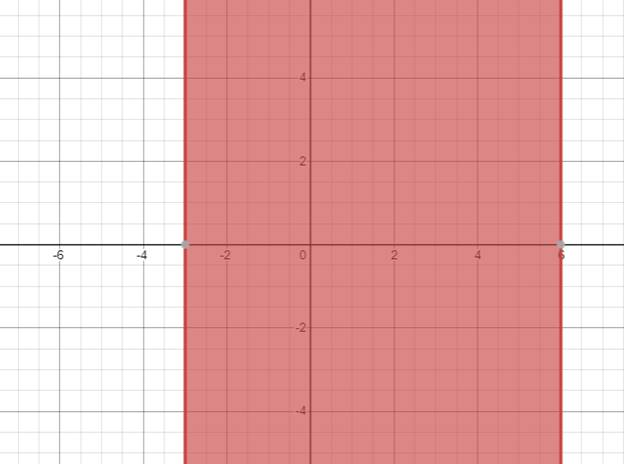# The solution of given nonlinear inequality equation and plot a graph for it.### Precalculus: Mathematics for Calcu...

6th Edition
Stewart + 5 others
Publisher: Cengage Learning
ISBN: 9780840068071### Precalculus: Mathematics for Calcu...

6th Edition
Stewart + 5 others
Publisher: Cengage Learning
ISBN: 9780840068071

#### Solutions

Chapter 1.7, Problem 39E
To determine

## The solution of given nonlinear inequality equation and plot a graph for it.

Expert Solution

3x6

### Explanation of Solution

Given:

Non-linear inequality equation

x23x180

Calculation,

Non-linear inequality equation,

x23x180x2(63)x180x26x+3x180x(x6)+3(x6)0(x+3)(x6)0Now,(x+3)0x3and,(x6)0x6so,3x6

Now, graph of x23x180Conclusion:

Hence, the values of x lies between the -3 and 6.

### Have a homework question?

Subscribe to bartleby learn! Ask subject matter experts 30 homework questions each month. Plus, you’ll have access to millions of step-by-step textbook answers!